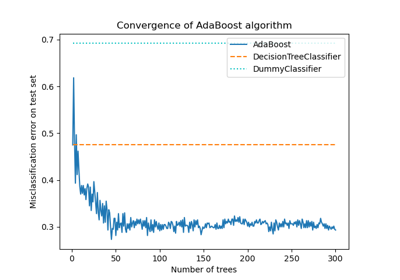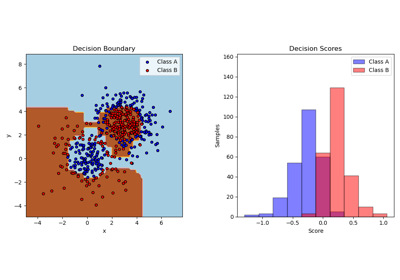# sklearn.datasets.make_gaussian_quantiles¶

sklearn.datasets.make_gaussian_quantiles(*, mean=None, cov=1.0, n_samples=100, n_features=2, n_classes=3, shuffle=True, random_state=None)[source]

Generate isotropic Gaussian and label samples by quantile.

This classification dataset is constructed by taking a multi-dimensional standard normal distribution and defining classes separated by nested concentric multi-dimensional spheres such that roughly equal numbers of samples are in each class (quantiles of the $$\chi^2$$ distribution).

Read more in the User Guide.

Parameters:
meanndarray of shape (n_features,), default=None

The mean of the multi-dimensional normal distribution. If None then use the origin (0, 0, …).

covfloat, default=1.0

The covariance matrix will be this value times the unit matrix. This dataset only produces symmetric normal distributions.

n_samplesint, default=100

The total number of points equally divided among classes.

n_featuresint, default=2

The number of features for each sample.

n_classesint, default=3

The number of classes.

shufflebool, default=True

Shuffle the samples.

random_stateint, RandomState instance or None, default=None

Determines random number generation for dataset creation. Pass an int for reproducible output across multiple function calls. See Glossary.

Returns:
Xndarray of shape (n_samples, n_features)

The generated samples.

yndarray of shape (n_samples,)

The integer labels for quantile membership of each sample.

Notes

The dataset is from Zhu et al .

References


1. Zhu, H. Zou, S. Rosset, T. Hastie, “Multi-class AdaBoost”, 2009.

## Examples using sklearn.datasets.make_gaussian_quantiles¶Plot randomly generated classification dataset

Plot randomly generated classification dataset